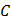# A cylindrical cavity of diameter a exists inside a cylinder of diametershown in the figure. Both the cylinder and the cavity are infinitely long. A uniform current densityflows along the length. If the magnitude of the magnetic field at the pointis given by, then the value ofis## Question ID - 153954 :- A cylindrical cavity of diameter a exists inside a cylinder of diametershown in the figure. Both the cylinder and the cavity are infinitely long. A uniform current densityflows along the length. If the magnitude of the magnetic field at the pointis given by, then the value ofis3537

 (5)Next Question :

A small coilwithturns is mounted on one end of a balance beam and introduced between the poles of an electromagnet as shown in the figure. The area of the coil is, the length of the right arm of the balance beam iscm . When there is no current in the coil the balance is in equilibrium. On passing a currentmAthrough the coil, equilibrium is restored by putting an additional weight of massmg on the balance pan. Find the magnetic induction field (in terms ofT) between the poles of the electromagnet, assuming it to be uniform